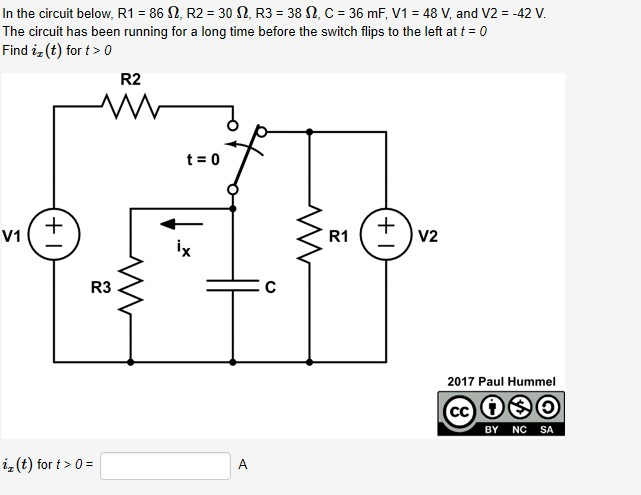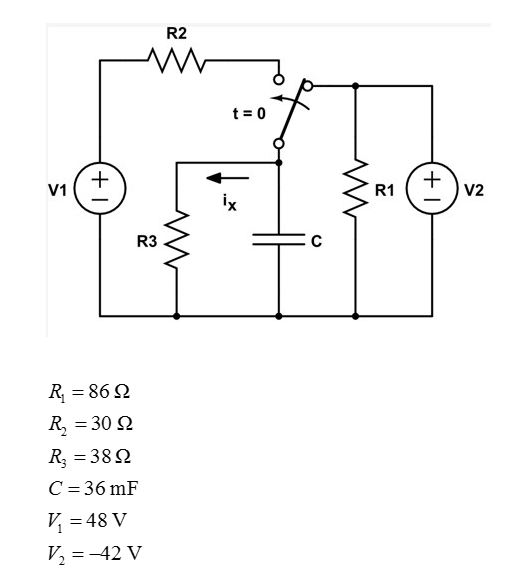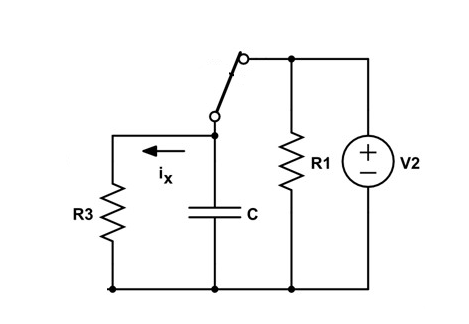# In the circuit below, R1 = 86 N, R2 = 30 N, R3 = 38 N, C = 36 mF, V1 = 48 V, and V2 = -42 V.The circuit has been running for a long time before the switch flips to the left at t = 0Find iz (t) for t> 0R2t = 0V2V1R1ixR32017 Paul HummelNCSABYiz(t) for t> 0 =A(+1

Question
2 viewshelp_outlineImage TranscriptioncloseIn the circuit below, R1 = 86 N, R2 = 30 N, R3 = 38 N, C = 36 mF, V1 = 48 V, and V2 = -42 V. The circuit has been running for a long time before the switch flips to the left at t = 0 Find iz (t) for t> 0 R2 t = 0 V2 V1 R1 ix R3 2017 Paul Hummel NC SA BY iz(t) for t> 0 = A (+1 fullscreen
check_circle

Step 1

The given circuit diagram and values of element is:Step 2

The modified circuit diagram at t = 0-:Step 3

The voltage across capacitor and the value of ix at t = 0- is determined as follows:

The value of capacitor voltage is equal to the supply voltage V2 because the circuit has been running for a long time before the switch flips.

...

### Want to see the full answer?

See Solution

#### Want to see this answer and more?

Solutions are written by subject experts who are available 24/7. Questions are typically answered within 1 hour.*

See Solution
*Response times may vary by subject and question.
Tagged in

### Electrical Engineering